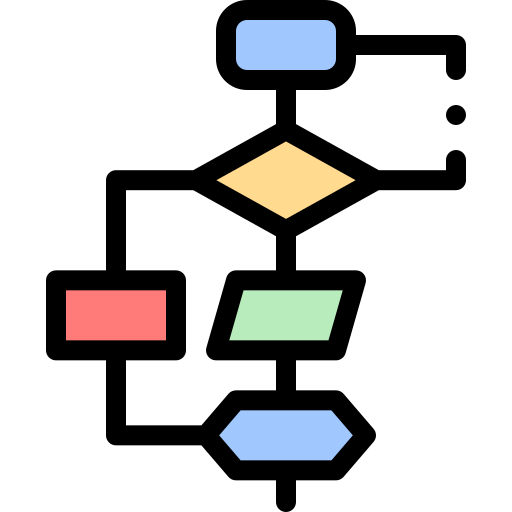演算法 : 時間複雜度-漸進符號 (Asymptotic notation)漸進符號有哪些

1. $O$ – notation (Big-oh)

2. $\Omega$ – notation (Big-omega)

3. $\Theta$ – notation (Big-theta)

4. $o$ – notation (Little-oh)

5. $\omega$ – notation (Little-omega)

1-3 這三個是最常使用的漸進符號，尤其是第一個 Big-Oh， 4-5 則幾乎不會用到。下面我會介紹這五個漸進符號的定義及例子。

Big-oh

$$O(g(n)) = { f(n): \exists c > 0, \ n_{0} > 0 \, \ s.t. \, 0 \leq f(n) \leq c \, g(n), \ \forall n \geq n_{0} }$$

1. $c, n_{0}$ 皆為正整數
2. $n = 5$ 時代入得 $f(5) = 30, \, 6 \times g(5) = 30$ 符合 $0 \leq f(n) \leq c \, g(n)$，繼續代 $n = 6, \, n = 7, \, \cdots$ 你會發現都將符合定義 （可參考下圖）<<Introduction to Algorithms>> 俗稱楓葉書或是 CLRS(作者名)由 Thomas H. Cormen, Charles E. Leiserson, Ronald L. Rivest, Clifford Stein 撰寫，是演算法的聖經書。聽說每個軟體工程師的包包裡面都會有一本。Grandpa